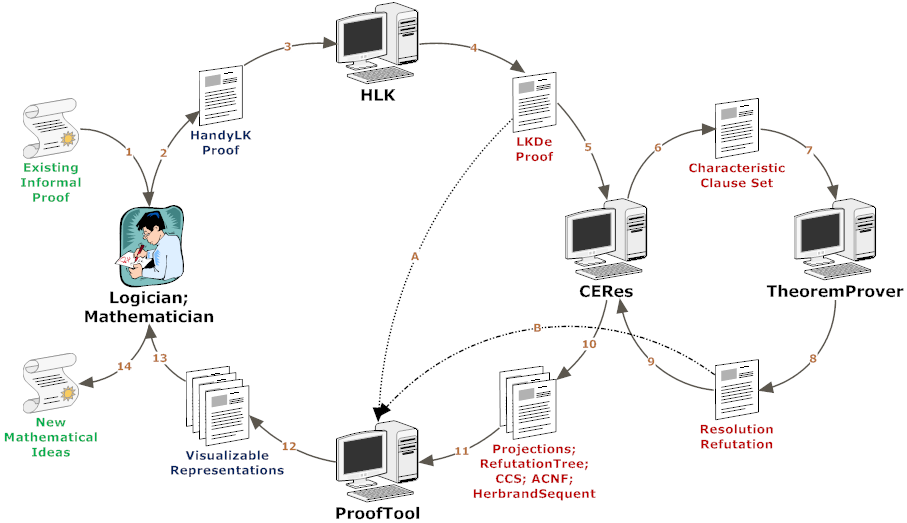TU Vienna logic.at Theory and Logic Group (E185-2)

## The System CERES

There are three main tasks:

• One is to compute the characteristic clause set CL(φ).
• The others are to generate a resolution refutation of CL(φ) by an external theorem prover. The current version of CERES uses the automated theorem prover Prover9, but any refutational theorem prover based on resolution and paramodulation like Otter or ATP may be used.
• And to compute the necessary projections of φ w.r.t. the clauses in CL(φ) actually used for the refutation. The properly instantiated projections are concatenated, using the refutation obtained by the theorem prover as a skeleton of a proof with only atomic cuts.

During theorem proving equality is treated by paramodulation; its application within the final clausal refutation is then transformed to the appropriate equality rules in LKDe.

The definition introductions do not require any other special treatment within CERES than all other unary rules; in particular, they have no influence on the theorem proving part.

Since the restriction to skolemized proofs is crucial to the CERES method, the system also performs skolemization (according to Andrews' method) on the input proof.

The following figure illustrates the typical workflow and dataflow when working with CERES:The system CERES expects an LKDe proof of a sequent S and a set of axioms as input, and, after validation of the input proof, computes a proof of sk(S) containing at most atomic-cuts. Input and output are formatted using the well known data representation language XML. This allows the use of arbitrary and well known utilities for editing, transformation and presentation and standardized programming libraries.

To increase the performance and avoid redundancy, most parts of the proofs are internally represented as directed acyclic graphs. This representation turns out to be very handy, also for the internal unification algorithms.

The formal analysis of mathematical proofs (especially by a mathematician as a pre- and post-"processor") relies on a suitable format for the input and output of proofs, and on an appropriate aid in dealing with them. We developed an intermediary proof language HLK (Handy LK) connecting the language of mathematical proofs with LKDe and the compiler transforming proofs written in this higher-level language to LKDe.

In general, writing down proofs in LK for automated processing or typesetting is usually done in a LISP like notation for tree structures. Such approaches result in deep nested constructs that become hardly manageable when the number of proof nodes grows. Another common problem is redundancy: each formula has traditionally many copies on the way from its introduction to its elimination (or to the end-sequent). In addition structural rules (except the cut) do not need to be particularized and, after all quantifiers have been introduced on a branch in the proof tree, the rules above this proof node are about propositional, i.e. they can be generated automatically. The goal of the language HLK is to minimize the input by applying the considerations discussed above.

Finally it should be noted, that HLK can handle meta-terms and meta-formulas in proofs, and is capable of translating recursive definitions of proof schemata, enabling the user to define infinite proof sequences.

Furthermore we implemented a proof tool with a graphical user interface, allowing a convenient input and analysis of the output of the system CERES. Thereby the integration of definition- and equality-rules into the underlying calculus plays an essential role in overlooking, understanding and analyzing complex mathematical proofs by humans.